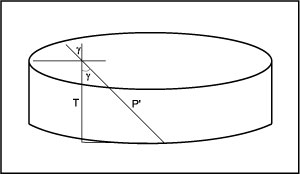ULYSSES

Ulysses HISCALE Data Analysis Handbook

4.12 Calculated Responses of HISCALE Sensors to Omnidirectionally Penetrating Cosmic Galactic Rays

Document Source: James Tappin and Dennis Haggerty

4.12.1 Numerical Simulation of HISCALE Response to Galactic Cosmic Rays

This simulation models isotropic flux through a cylindrical detector. The dimensions of the detector are: radius = 5652 mm, thickness = 200 mm. The simulation determines the distance traveled in the detector for each randomly entering particle. For clarity the results have been placed in three separate categories. They are:

Case 1 - Top entering particles leaving through the detector side.
Case 2 - Top entering particles leaving through the bottom.
Case 3 - Side entering particles leaving through a side.

The energy deposition due to these particles can be determined by the equation:

Energy = 0.466 keV/Micron * Penetration distance

The slope of the graph is approximately 3.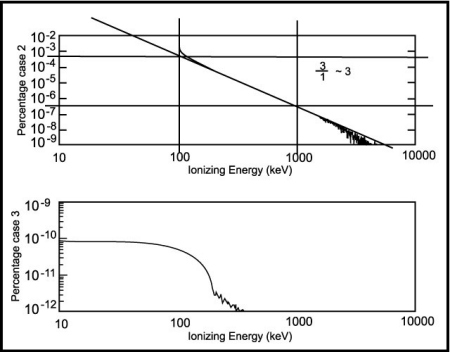Figure 4.85 Cosmic ray case 1 (oblique) and total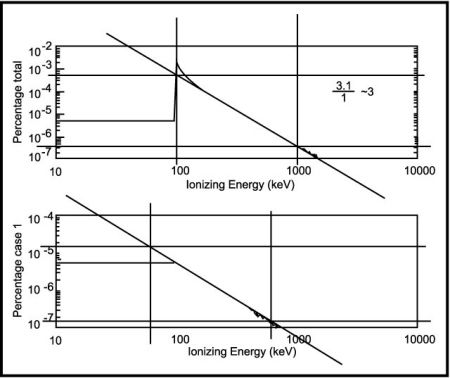Figure 4.86 Calculations of penetration geometry, part Itan g=L/T L=T tan g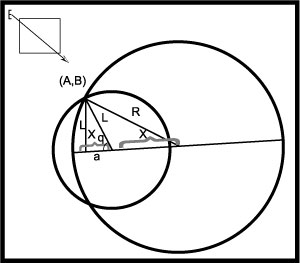A=L cos q B=L sin q A=T tan g cos q B=T tan g sin q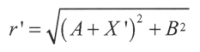If r' > r             case 1 Else r' <= r      case 2

Figure 4.87 Calculations of penetration geometry, part II

CASE 1: Projection through side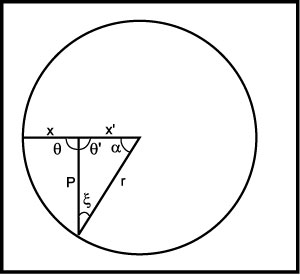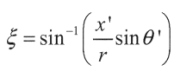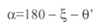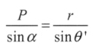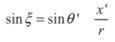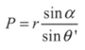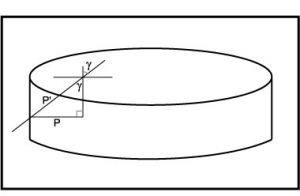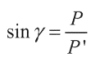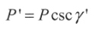CASE 2: Projection through bottom